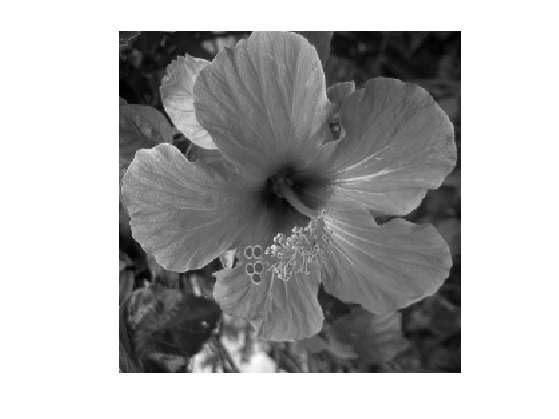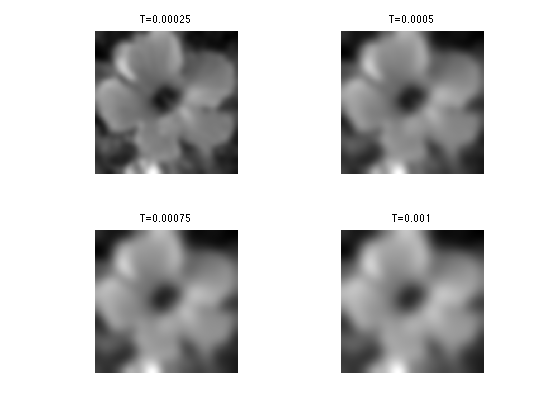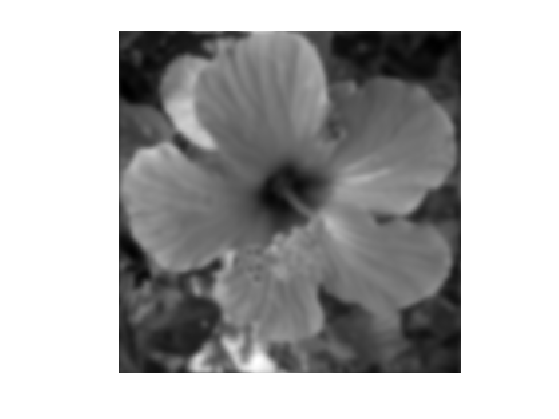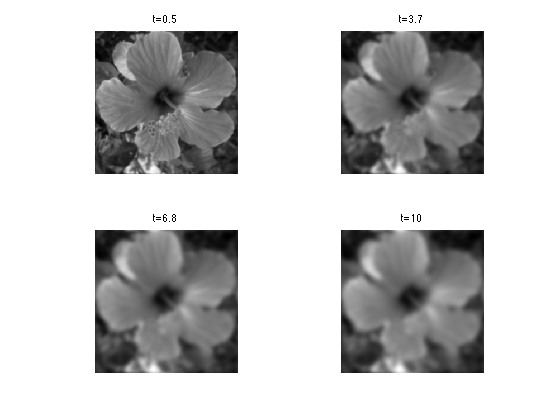$\newcommand{\NN}{\mathbb{N}} \newcommand{\CC}{\mathbb{C}} \newcommand{\GG}{\mathbb{G}} \newcommand{\LL}{\mathbb{L}} \newcommand{\PP}{\mathbb{P}} \newcommand{\QQ}{\mathbb{Q}} \newcommand{\RR}{\mathbb{R}} \newcommand{\VV}{\mathbb{V}} \newcommand{\ZZ}{\mathbb{Z}} \newcommand{\FF}{\mathbb{F}} \newcommand{\KK}{\mathbb{K}} \newcommand{\UU}{\mathbb{U}} \newcommand{\EE}{\mathbb{E}} \newcommand{\Aa}{\mathcal{A}} \newcommand{\Bb}{\mathcal{B}} \newcommand{\Cc}{\mathcal{C}} \newcommand{\Dd}{\mathcal{D}} \newcommand{\Ee}{\mathcal{E}} \newcommand{\Ff}{\mathcal{F}} \newcommand{\Gg}{\mathcal{G}} \newcommand{\Hh}{\mathcal{H}} \newcommand{\Ii}{\mathcal{I}} \newcommand{\Jj}{\mathcal{J}} \newcommand{\Kk}{\mathcal{K}} \newcommand{\Ll}{\mathcal{L}} \newcommand{\Mm}{\mathcal{M}} \newcommand{\Nn}{\mathcal{N}} \newcommand{\Oo}{\mathcal{O}} \newcommand{\Pp}{\mathcal{P}} \newcommand{\Qq}{\mathcal{Q}} \newcommand{\Rr}{\mathcal{R}} \newcommand{\Ss}{\mathcal{S}} \newcommand{\Tt}{\mathcal{T}} \newcommand{\Uu}{\mathcal{U}} \newcommand{\Vv}{\mathcal{V}} \newcommand{\Ww}{\mathcal{W}} \newcommand{\Xx}{\mathcal{X}} \newcommand{\Yy}{\mathcal{Y}} \newcommand{\Zz}{\mathcal{Z}} \newcommand{\al}{\alpha} \newcommand{\la}{\lambda} \newcommand{\ga}{\gamma} \newcommand{\Ga}{\Gamma} \newcommand{\La}{\Lambda} \newcommand{\Si}{\Sigma} \newcommand{\si}{\sigma} \newcommand{\be}{\beta} \newcommand{\de}{\delta} \newcommand{\De}{\Delta} \renewcommand{\phi}{\varphi} \renewcommand{\th}{\theta} \newcommand{\om}{\omega} \newcommand{\Om}{\Omega} \renewcommand{\epsilon}{\varepsilon} \newcommand{\Calpha}{\mathrm{C}^\al} \newcommand{\Cbeta}{\mathrm{C}^\be} \newcommand{\Cal}{\text{C}^\al} \newcommand{\Cdeux}{\text{C}^{2}} \newcommand{\Cun}{\text{C}^{1}} \newcommand{\Calt}{\text{C}^{#1}} \newcommand{\lun}{\ell^1} \newcommand{\ldeux}{\ell^2} \newcommand{\linf}{\ell^\infty} \newcommand{\ldeuxj}{{\ldeux_j}} \newcommand{\Lun}{\text{\upshape L}^1} \newcommand{\Ldeux}{\text{\upshape L}^2} \newcommand{\Lp}{\text{\upshape L}^p} \newcommand{\Lq}{\text{\upshape L}^q} \newcommand{\Linf}{\text{\upshape L}^\infty} \newcommand{\lzero}{\ell^0} \newcommand{\lp}{\ell^p} \renewcommand{\d}{\ins{d}} \newcommand{\Grad}{\text{Grad}} \newcommand{\grad}{\text{grad}} \renewcommand{\div}{\text{div}} \newcommand{\diag}{\text{diag}} \newcommand{\pd}{ \frac{ \partial #1}{\partial #2} } \newcommand{\pdd}{ \frac{ \partial^2 #1}{\partial #2^2} } \newcommand{\dotp}{\langle #1,\,#2\rangle} \newcommand{\norm}{|\!| #1 |\!|} \newcommand{\normi}{\norm{#1}_{\infty}} \newcommand{\normu}{\norm{#1}_{1}} \newcommand{\normz}{\norm{#1}_{0}} \newcommand{\abs}{\vert #1 \vert} \newcommand{\argmin}{\text{argmin}} \newcommand{\argmax}{\text{argmax}} \newcommand{\uargmin}{\underset{#1}{\argmin}\;} \newcommand{\uargmax}{\underset{#1}{\argmax}\;} \newcommand{\umin}{\underset{#1}{\min}\;} \newcommand{\umax}{\underset{#1}{\max}\;} \newcommand{\pa}{\left( #1 \right)} \newcommand{\choice}{ \left\{ \begin{array}{l} #1 \end{array} \right. } \newcommand{\enscond}{ \left\{ #1 \;:\; #2 \right\} } \newcommand{\qandq}{ \quad \text{and} \quad } \newcommand{\qqandqq}{ \qquad \text{and} \qquad } \newcommand{\qifq}{ \quad \text{if} \quad } \newcommand{\qqifqq}{ \qquad \text{if} \qquad } \newcommand{\qwhereq}{ \quad \text{where} \quad } \newcommand{\qqwhereqq}{ \qquad \text{where} \qquad } \newcommand{\qwithq}{ \quad \text{with} \quad } \newcommand{\qqwithqq}{ \qquad \text{with} \qquad } \newcommand{\qforq}{ \quad \text{for} \quad } \newcommand{\qqforqq}{ \qquad \text{for} \qquad } \newcommand{\qqsinceqq}{ \qquad \text{since} \qquad } \newcommand{\qsinceq}{ \quad \text{since} \quad } \newcommand{\qarrq}{\quad\Longrightarrow\quad} \newcommand{\qqarrqq}{\quad\Longrightarrow\quad} \newcommand{\qiffq}{\quad\Longleftrightarrow\quad} \newcommand{\qqiffqq}{\qquad\Longleftrightarrow\qquad} \newcommand{\qsubjq}{ \quad \text{subject to} \quad } \newcommand{\qqsubjqq}{ \qquad \text{subject to} \qquad }$

Linear Diffusion Flows

# Linear Diffusion Flows

This tours studies linear diffusion PDEs, a.k.a. the heat equation. A good reference for diffusion flows in image processing is [Weickert98].

## Installing toolboxes and setting up the path.

You need to download the following files: signal toolbox and general toolbox.

You need to unzip these toolboxes in your working directory, so that you have toolbox_signal and toolbox_general in your directory.

For Scilab user: you must replace the Matlab comment '%' by its Scilab counterpart '//'.

Recommandation: You should create a text file named for instance numericaltour.sce (in Scilab) or numericaltour.m (in Matlab) to write all the Scilab/Matlab command you want to execute. Then, simply run exec('numericaltour.sce'); (in Scilab) or numericaltour; (in Matlab) to run the commands.

Execute this line only if you are using Matlab.

getd = @(p)path(p,path); % scilab users must *not* execute this


Then you can add the toolboxes to the path.

getd('toolbox_signal/');
getd('toolbox_general/');


## Heat Diffusion

The heat equation reads $\forall t>0, \quad \pd{f_t}{t} = \nabla f_t$ for a function $$f_t : \RR^2 \rightarrow \RR$$ and where $$f_0$$ (the solution at initial time $$t=0$$) is given.

The Laplacian operator reads $\Delta f = \pdd{f}{x_1} + \pdd{f}{x_2}.$

The flow is discretized in space by considering a discrete image of $$N = n \times n$$ pixels.

n = 256;


Load an image $$f_0 \in \RR^N$$, that will be used to initialize the flow at time $$t=0$$.

name = 'hibiscus';
f0 = rescale( sum(f0,3) );


Display it.

clf;
imageplot(f0);The flow is discretized in time using an explicit time-stepping $f^{(\ell+1)} = f^{(\ell)} + \tau \Delta f^{(\ell)}.$ We use finite difference Laplacian $(\Delta f)_i = \frac{1}{h^2}\pa{ f_{i_1+1,i_2}+f_{i_1-1,i_2}+f_{i_1,i_2+1}+f_{i_1,i_2-1}-4f_j }$ where we assume periodic boundary conditions, and where $$h = 1/N$$ is the spacial step size.

h = 1/n;


The step size $$\tau$$ should satisfy $\tau < \frac{h^2}{4}$ for the discretized flow to be stable.

The discrete solution $$f^{(\ell)}$$ converges to the continuous solution $$f_t$$ at time $$t = \tau \ell$$ if both $$\tau \rightarrow 0$$ and $$h \rightarrow 0$$ under the condition $$\tau/h^2 < 1/4$$.

Select a small enough step size.

tau = .5 * h^2/4;


Final time.

T = 1e-3;


Number of iterations.

niter = ceil(T/tau);


Initialize the diffusion at time $$t=0$$.

f = f0;


One step of discrete diffusion.

f = f + tau * delta(f);


Exercice 1: (check the solution) Compute the solution to the heat equation.

exo1;## Explicit Solution using Convolution

The solution to the heat equation can be computed using a convolution $\forall t>0, \quad f_t = f_0 \star h_t$ where $$\star$$ denotes the convolution of continuous functions $f \star h(x) = \int_{\RR^2} f(y) g(x-y) d y$ and $$h_t$$ is a Gaussian kernel of width $$\sqrt{t}$$ $h_t(x) = \frac{1}{4 \pi t} e^{ -\frac{\norm{x}^2}{4t} }$

One can thus approximate the solution using a discrete convolution. Convolutions can be computed in $$O(N\log(N))$$ operations using the FFT, since $g = f \star h \qarrq \forall \om, \quad \hat g(\om) = \hat f(\om) \hat h(\om).$

cconv = @(f,h)real(ifft2(fft2(f).*fft2(h)));


Define a discrete Gaussian blurring kernel of width $$\sqrt{t}$$.

t = [0:n/2 -n/2+1:-1];
[X2,X1] = meshgrid(t,t);
normalize = @(h)h/sum(h(:));
h = @(t)normalize( exp( -(X1.^2+X2.^2)/(4*t) ) );


Define blurring operator.

heat = @(f, t)cconv(f,h(t));


Example of blurring.

clf;
imageplot(heat(f0,2));Exercice 2: (check the solution) Display the heat convolution for increasing values of $$t$$.

exo2;